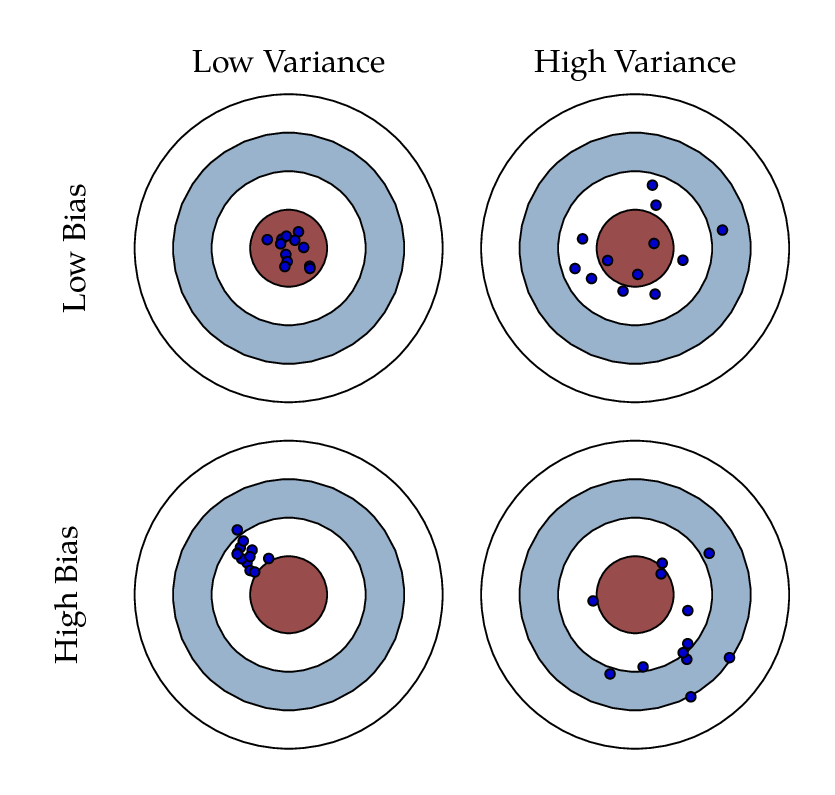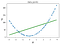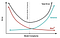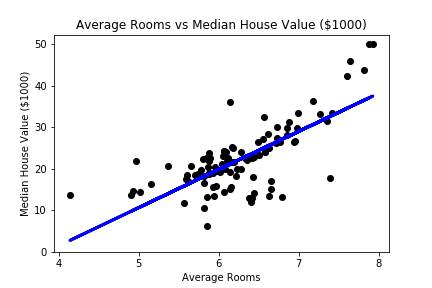# The Bias-Variance Tradeoff

If you are going to study data science, data analysis, machine learning, or any other discipline that builds models to make predictions on data, you are going to stumble upon the term “Bias-Variance Tradeoff”. Basically, it describes the tradeoff of a model learning “too much” or “too little” in the training process. If a model learns the training data too much, it will perform weakly on data is has not seen (the test data). If a model does not learn enough, it will perform poorly on all data because it has not captured the trend it has been created to capture.

To visualize the bias-variance tradeoff image the game of darts, and since we are not professional the goal will be to hit the center dot. Bias is going to represent how far we are from hitting the center with our set of darts. Variance is how different in location each of our darts is depending on the throw.Figure 1

With some research you will find that there are a couple definitions of bias and variance in the terms of modeling. I am going to list the one I find to be the most understandable and most accurate.

In this article by machine learning mastery (an incredible resource) he has the following definitions (denoted with quotation marks):

Bias are the simplifying assumptions made by a model to make the target function easier to learn.” The word assumptions here means the decisions the model has made in order for that algorithm to best predict (based on some score) the target function.

High-bias will occur if we do not provide enough information to the model, and without enough information it cannot capture the relationship between the features and the target. This model will be under-fit, and the predictions will be inaccurate on all prediction (as you can see in the bottom row of figure 1). For example, if we are trying to capture a non-linear trend with a linear function it will never be able to really capture the trend due to the nature of the function.

High-bias machine learning algorithms are those that try to model the target function. These include linear regression and logistic regression. Looking at figure 2, the linear regression model (the line) has made an assumption as to what the true target function is, and in this case is very inaccurate due to a line (linear function) not being able to model a curve (exponential function). This does not mean that they will be inaccurate on every application, but due to the nature of the algorithm are more susceptible for high bias.Figure 2 [Source]

Low-Bias will occur when we provide a sufficient amount of information to the model and it is able to predict accurately (as you can see in the top row of figure 1). For example, if weight is highly correlated to the number of calories eaten on average, we will be able to predict weight very accurately if average caloric intake is a feature.

Low-bias machine learning algorithms are those that do not try and model the target function. Their algorithms are able to model the target in different ways. These include decision trees, k-nearest neighbors, and support vector machines. In a decision tree, there are no assumptions of the target, just smaller decision to try and split the data based on some criteria (usually gini or entropy) that will lead to good predictions.

Variance is the amount that the estimate of the target function will change if different training data was used.” If you were to take a different train-test split, how much would that model differ?

High-variance will occur if we allow the model to learn too much about the data that it is training on. The model will learn the noise within the data and will not understand the trend we are trying to capture, but has essentially memorized the training data. This will lead to exceptionally good results on the training data and very poor results on the test data. In terms of our definition the model will change vastly depending the on the training data it received. This does not mean that these algorithms will perform poorly, but that they are more susceptible for it.

High-variance machine learning algorithms are those that will change drastically depending on the training data it receives. These include decision trees, k-nearest neighbors, and support vector machines. In a decision tree if it happens to not get a certain piece of data, a decision node that was previously made for that piece of data may never be made due to the lack of that data. The decision tree will change drastically because the decisions it makes are based on the data it received and not trends it is able to identify in the data.

Low-variance will occur if the model will make the same assumptions and create a similar model independent of the data it receives.

Low-variance machine learning algorithms are those that will not change when it receives different data for training. These include linear regression and logistic regression. If you look a linear regression line, even if you were to move a lot of those points around (still adhering to the target function) the algorithm would still create a very similar line.Bias-Variance Tradeoff

The reason this is a tradeoff is in order for one to go down the other must go up. It is the balancing act of having a model that is complex enough to capture the desired trend but not too complex where it is capturing the noise within the data. The equation for error within a model is `Total Error = Prediction Error + Irreducible Error` which can be further broken down to `Total Error = (Model Bias)^2 + Model Variance + Irreducible Error`. Irreducible error is the noise within the data that no model can capture. As you can see though, the bias term is squared making the same change in variance and bias have a different effect on the total error. There is no algorithm or one size fits all solution, but knowing this and adding things like early stopping can allow a model to be just the right amount of complex.

# Showing this experimentally

In order to show bias experimentally I will performing linear regression upon the Boston housing dataset included with `sklearn.datasets`. Linear regression is categorized as a high bias machine learning algorithm because it makes an assumption as to the weight of each feature on the target variable. For example, linear regression for predicting house prices using number of bedrooms might assign a weighting of 10,000 dollars. This means that for every additional bedroom, the cost of the house will increase 10,000 dollars.

Here is the code for linear regression using average number of rooms to predict the median value of that house.

From this code the model has made an assumption that for every additional room in a home, the price increase \$8,639. The assumption it has made is the best it can get to modeling the target function but with a root mean squared error (RMSE) of 6.75 on the test set we can see this is quite inaccurate. I will do this with 3 different random states to show that the assumptions it makes will be very similar despite different training and test sets.3 Different Random State [Figure 3]

As we can see in figure 3, the relationship between the number of rooms and the median value of the house is somewhat linear, but not exactly. From our house prices we can see that as the number of houses increases it is not linear but almost exponential, seeing a more drastic increase from 7–8 as seen from 6–7. This shows a high bias due to being generally incorrect despite the different training data and models.

In order to show variance experimentally I will create a decision tree in order to predict the class in the well known iris dataset. Decision trees are known to have high variance because it may make drastically different decision nodes based on the training data it receives. This will be much easier seen in the follow decision trees.

Here is the code for a decision tree using sepal length (cm), sepal width (cm), petal length (cm), and petal width (cm) to predict the class of that iris plant. The 3 different classifications are setosa, versicolor, virginica.

From this code the model performed amazing with accuracy ranging from 93%–96%. The trees created however, were quite different in their formation.

Trees 1 and 2 first split on feature 3 while Tree 3 split on feature 2. This due to the variance in decision trees and how their formation is completely dependent on the training data it receives.

This balancing act can be hard and gets easier with practice of training machine learning models. There is a lot you can do to make a model more complex or less complex combatting either bias or variance. In the end though, there is no perfect model and finding good-enough can sometimes be the goal.

If you have any questions, comments, or concerns please feel free to reach out.

Soon to be data scientist! I also love shoes and my dogs!

## More from Justin Fernandez

Soon to be data scientist! I also love shoes and my dogs!